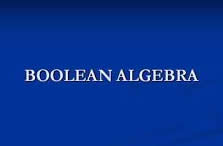# Difference between Boolean and Binary

##### Key Difference: In the field of computers and electronics, Boolean refers to a data type that has two possible values representing true and false. It is generally used in context to a deductive logical system known as Boolean Algebra. Binary in mathematics and computers, refers to a base 2 numerical notation. It consists of two values 0 and 1. The digits are combined using a place value structure to generate equivalent numerical values. Thus, both are based on the same underlying concept but used in context to different systems.Booelan denotes a system of algebric notation that was created by George Boole. The system can be defined by the occurrence of two states – True or False. 1(yes/on) are regarded equivalent to true, whereas 0 (no/off) are regarded as equivalent to false. Thus, we can say that Boolean Algebra is based on a binary (two values) system. It uses operators to determine the comparison between the bits. The most often used operators are AND and OR.Generally the word, binary is associated with two states know as 0 and 1. In mathematics, it specifically refers to base two arithmetic that uses the digits 0 and 1, therefore, the arithmetic is also defined as the binary arithmetic. Thus, we can say that Boolean values are represented with the notation of binary digits. Therefore, many times Boolean algebra is also known as the binary logic. However, Binary system is used to denote a system that is expressed in numerical notation that has base 2.

Comparison between Boolean and Binary:

 Boolean Binary Definition In the field of computers and electronics, Boolean refers to a data type that has two possible values representing true and false. It is generally used in context to a deductive logical system known as Boolean Algebra. Binary in Mathematics and computers, refers to a base 2 numerical notation. It consists of two values 0 and 1. The digits are combined using a place value structure to generate equivalent numerical values. Origin Named after George Boole (1815-1864) The term binary from Late Latin binarius "consisting of two" Method of Usage There are 4 main boolean operators: AND, NOT, OR, and XOR. x AND y returns True if both x and y are true, otherwise the expression returns False. NOT x returns True if x is false (or null) and False if x is true. x OR y returns True if either x or y or both are true; only if they are both false will it return False. x XOR y returns True if either x or y are true, but not both. If x and y are both true or false, the statement will return False. A binary number system is also called as base-2 number system. Addition - For example, 1 + 1 + 1 = 3 in base 10 becomes 1 + 1 + 1 = 11 in binary. Substraction - For example,  3 – 1 = 2 in base 10 becomes 11 – 1 = 10 in binary. Binary multiplication uses the same technique as decimal multiplication. To perform binary division follow the following steps: Step 1 - Align the divisor (Y) with the most significant end of the dividend. Let the portion of Step 2 - The dividend from its MSB to its bit aligned with the LSB of the divisor be denoted X. Step 3 - Compare X and Y. a) If X >= Y, the quotient bit is 1 and perform the subtraction X-Y. b) If X < Y, the quotient bit is 0 and do not perform any subtractions. Step 4 - Shift Y one bit to the right and go to step 2. Example Boolean expression can be denoted by an expression that results in a value of either TRUE or FALSE. For example, the expression 4 < 5 (4 is less than 5), is a Boolean expression as the result is always true for this particular statement. Decimal representation of a binary number - 100100 = [ ( 1 ) × 2^5 ] + [ ( 0 ) × 2^4 ] + [ ( 0 ) × 2^3 ] + [ ( 1 ) × 2^2 ] + [ ( 0 ) × 2^1 ] + [ ( 0 ) × 2^0 ] = 36

Image Courtesy: authorstream.com, waitingforfriday.com